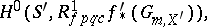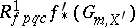User:Maximilian Janisch/latexlist/Algebraic Groups/Picard scheme

A natural generalization of the concept of the Picard variety $F ( X )$ for a smooth algebraic variety $x$ within the framework of the theory of schemes. To define the Picard scheme for an arbitrary $5$-scheme $x$ one considers the relative Picard functor $PICX / S$ in the category $\operatorname { sch } / S$ of schemes over the scheme $5$. The value of this functor on an $5$-scheme $S ^ { \prime }$ is the groupwhere $f ^ { \prime } : X \times s S ^ { \prime } \rightarrow S ^ { \prime }$ is the base-change morphism andis the sheaf in the Grothendieck topology $S _ { f } ^ { \prime } p q c$ of strictly-flat quasi-compact morphisms associated with the pre-sheaf

\begin{equation} T \rightarrow H ^ { 1 } ( T _ { f } p q c , G _ { m } ) = H ^ { 1 } ( T _ { et } , G _ { m } ) \end{equation}

and $G _ { m }$ denotes the standard multiplicative group sheaf. If the Picard functor $PICX / S$ is representable on $\operatorname { sch } / S$, then the $5$-scheme representing it is called the relative Picard scheme for the $5$-scheme $x$ and is denoted by $x / S$. If $x$ is an algebraic scheme over a certain field $k$ having a rational $k$-point, then

\begin{equation} \operatorname { Pic } _ { X / k } ( S ^ { \prime } ) = \operatorname { Fic } ( X \times k S ^ { \prime } ) / \operatorname { Fic } ( S ^ { \prime } ) \end{equation}

for any $k$-scheme $S ^ { \prime }$ . In particular, $k ( k ) = \operatorname { Pic } ( X )$ can be identified with the group of $k$-rational points $\operatorname { Pic } _ { X / k } ( k )$ of $PICX / K$ if such exists.

If $f : X \rightarrow S$ is a projective morphism with geometrically-integral fibres, then the scheme $x / S$ exists and is a locally finitely representable separable group $5$-scheme. If $S = \operatorname { Spec } ( k )$, then the connected component of the unit, $\operatorname { Pic } _ { X / k } ^ { 0 }$, of $x / k$ is an algebraic $k$-scheme, and the corresponding reduced $k$-schemeis precisely the Picard variety $P _ { c } ( X )$ . The nilpotent elements in the local rings of the scheme $\operatorname { Pic } _ { X / k } ^ { 0 }$ give much additional information on the Picard scheme and enable one to explain various "pathologies" in algebraic geometry over a field of characteristic $p > 0$. On the other hand, over a field of characteristic 0 the scheme $\operatorname { Pic } _ { K / k } ^ { Q }$ is always reduced . It is also known that $PICF / k$ is a reduced scheme if $H ^ { \prime }$ is a smooth algebraic surface and $H ^ { 2 } ( F , O _ { F } ) = 0$ .

For any proper flat morphism $f : X \rightarrow S$ (finitely representable if the base $5$ is Noetherian) for which $f ^ { \prime } ( O _ { X ^ { \prime } } ) = O _ { S ^ { \prime } }$, the functor $PICX / S$ is an algebraic space over $5$ for any base-change morphism $f ^ { \prime } : X ^ { \prime } = X \times S S ^ { \prime } \rightarrow S$ . In particular, the functor $PICX / S$ is representable if the ground scheme $5$ is the spectrum of a local Artinian ring.

How to Cite This Entry:
Maximilian Janisch/latexlist/Algebraic Groups/Picard scheme. Encyclopedia of Mathematics. URL: http://encyclopediaofmath.org/index.php?title=Maximilian_Janisch/latexlist/Algebraic_Groups/Picard_scheme&oldid=44043